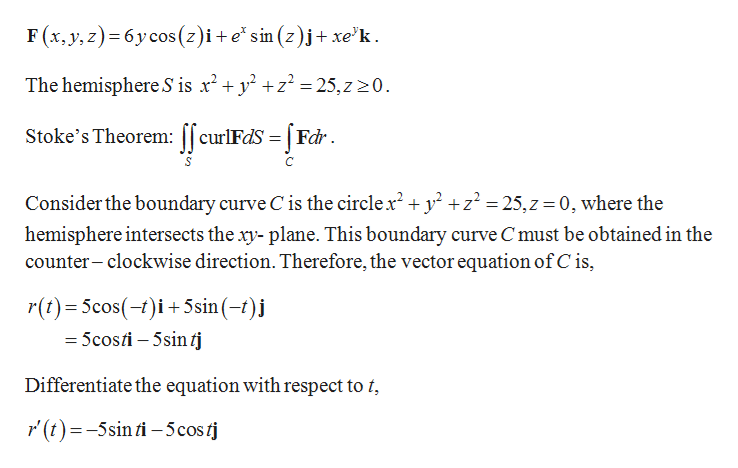# Use Stokes' Theorem to evaluate curl F · dS.F(x, y, z) = 6y cos(z) i + ex sin(z) j + xey k,S is the hemisphere x2 + y2 + z2 = 25, z ≥ 0, oriented upward.

Question
156 views

Use Stokes' Theorem to evaluate

curl F · dS.
F(x, y, z) = 6y cos(z) i + ex sin(z) j + xey k,

S is the hemisphere x2 + y2 + z2 = 25, z ≥ 0, oriented upward.
check_circle

Step 1

From the given s...help_outlineImage TranscriptioncloseF(x,y, z) 6y cos (z)i+esin (z)j+ xe'k. The hemisphere S is r2 225,z20 Stoke's Theorem:curlFdS =Fdr Consider the boundary curve C is the circlex2 + y2 z2 = 25,z 0, where the hemisphere intersects the xy- plane. This boundary curve C must be obtained in the counter-clockwise direction. Therefore, the vector equation of C is, r(t) 5cos(-)i5sin(-t) =5costi 5sinfj Differentiate the equation with respect to t (t)-5sinfi-5costj fullscreen

### Want to see the full answer?

See Solution

#### Want to see this answer and more?

Solutions are written by subject experts who are available 24/7. Questions are typically answered within 1 hour.*

See Solution
*Response times may vary by subject and question.
Tagged in

### Math